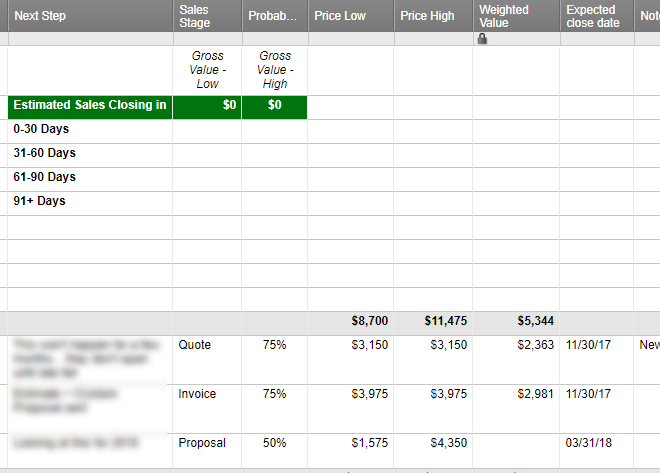#### Welcome to the Smartsheet Forum Archives

The posts in this forum are no longer monitored for accuracy and their content may no longer be current. If there's a discussion here that interests you and you'd like to find (or create) a more current version, please Visit the Current Forums.

# Pending Report - Formula Assistance Needed

On our sales tracking sheet, we track probability of the sale closing, expected close date, and the price low and price high of each potential sale. I'm currently using a modified version of the Sales Pipelne with Summary template.

In addition to the summary (that shows potential sales at every stage of the sales process), I want to create a chart at the top of each page that tracks the total amount of potential sales (the low price and the high price) that have a 51% or higher chance of closing. I want to show sales at that level of probability that are with 30 days of the expected close date. Then 31-60 days. Then 61-90 days.

We also track CLOSED/WON sales (at 100%) on this page, so if it is marked at 100%, I do not want to include it in the totals.

Basically, I need the right formula to capture this:

In the "Gross Value Low" column, on the first line (0-30 days), I need the SUM of all PRICE LOW of all entries if PROBABILITY is >50% but <100% AND the expected close date is 0-30 days away. Then I'd need the sum of the PRICE HIGH for those same conditions.

On the second line, in the "Gross Value Low" column, I'd need: the SUM of all PRICE LOW of all entries if PROBABILITY is >50% but <100% AND the expected close date is 31-60 days away.

And so on.

Can anyone help?Tags:

• Hi Cecily,

Try using SUMIFS formulas, one in your price low column and one in your price high column:

=SUMIFS([Price Low]:[Price Low], [Probability]:[Probability], >0.5, [Probability]:[Probability], <1)

Change the cell references as needed. If you need to reference a specific range, add the row numbers to the cell references, e.g. =SUMIFS([Price Low]7:[Price Low]15...)

• Hi Shaine,

That worked for giving me the sum of the Price Lows that had a probability higher than 50% but less than 100% -- thank you!

Now I need to add one more criterion: I need that same sum, but only If today's date is 0-30 days from the expected close date.

Or if today's date is 31-60 days from the expected close date.

How do I add that condition?

• I found a workaround, by adding a "net days" column and using that for the additional criterion.